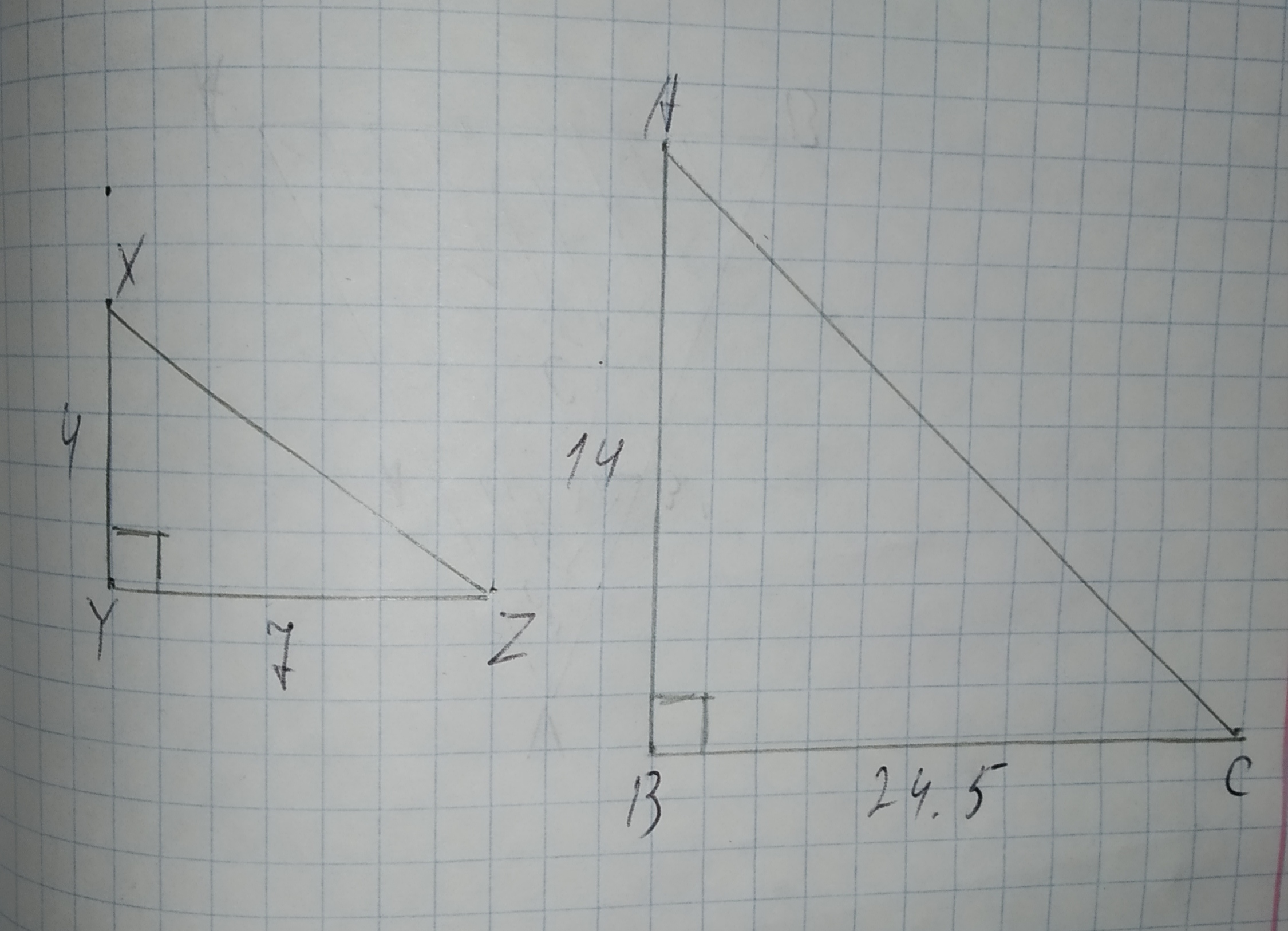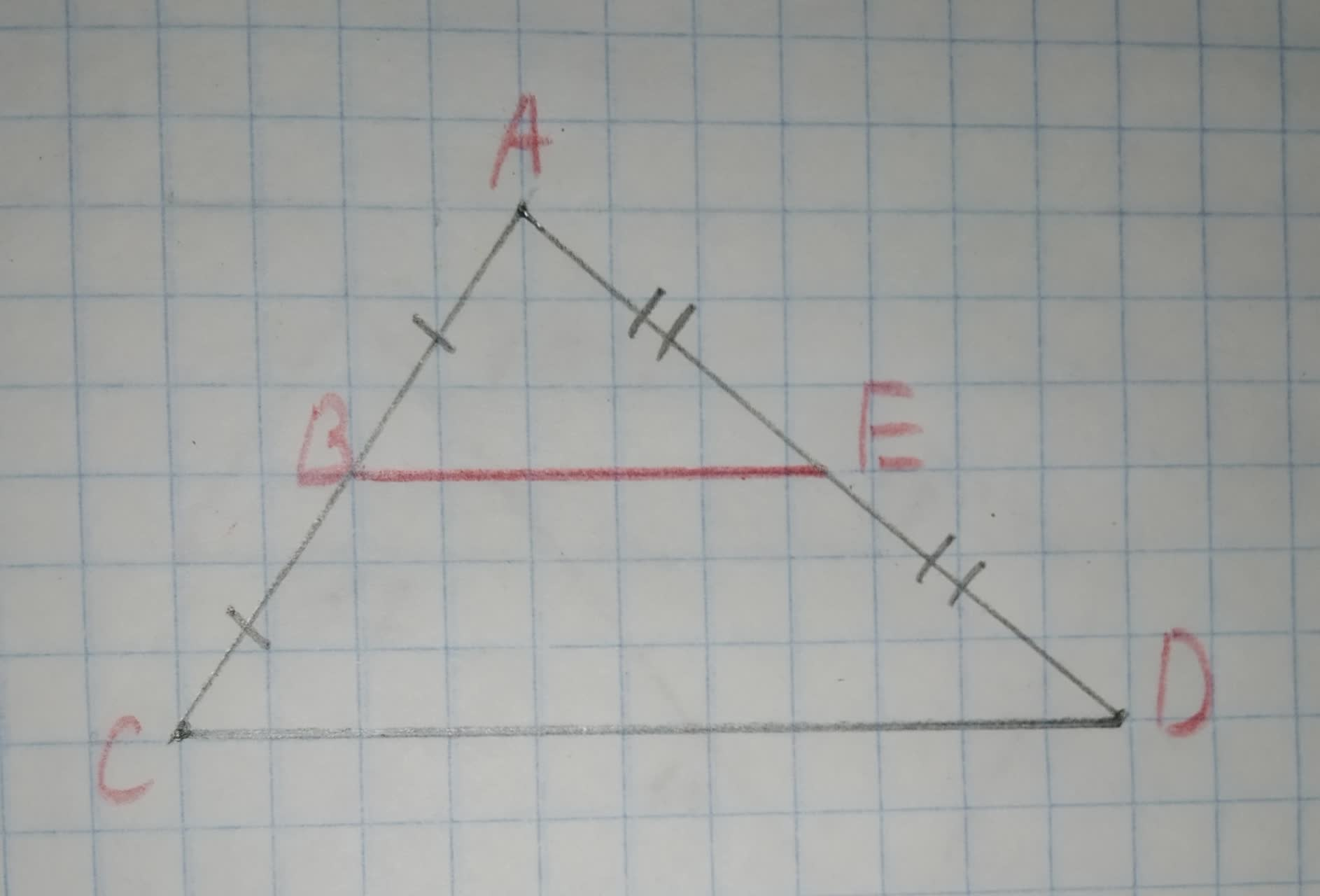# To determine: The value of segment HF Given information: The following information has been given EH=7 HG=4Question
SimilarityTo determine: The value of segment HF
Given information:
The following information has been given
EH=7
HG=42020-11-21
Formula used:
Similar triangles have sides in same ratio.
Calculation:
We know that in similar triangles HFE and FGE,
$$\displaystyle\frac{{{H}{F}}}{{{F}{G}}}=\frac{{{F}{E}}}{{{G}{E}}}=\frac{{{H}{E}}}{{{F}{E}}}$$(by law of similarity)
Hence, we substitute the value of EH and HG in the equation above , we get
$$\displaystyle{F}{E}^{{2}}={H}{E}{\left({G}{E}\right)}$$
Thus, we get
$$\displaystyle{F}{E}=\sqrt{{{77}}}$$

### Relevant QuestionsTo determine: The value of segment HF
Given information:
The following information has been given
EH=7
HG=3To determine: The value of segment HF
Given information:
The following information has been given
GF=6
EG=9To determine:To prove:The congruency of $$\displaystyle\angle{P}{B}{C}\stackrel{\sim}{=}\angle{P}{B}{D}$$.
Given information:
The following information has been given
$$\displaystyle\angle{B}{P}{C}=\angle{B}{P}{D}={90}^{{\circ}}$$
$$\displaystyle\angle{C}=\angle{D}$$To prove:The congruency of $$\displaystyle\vec{{{M}{H}}}\stackrel{\sim}{=}\vec{{{J}{O}}}$$.
Given information:
The following information has been given GJKM is a rhombus
$$\displaystyle\angle{J}{O}{G}=\angle{M}{H}{G}={90}^{{\circ}}$$To prove:The congruency of $$\displaystyle\vec{{{S}{O}}}\stackrel{\sim}{=}\vec{{{T}{O}}}$$.
Given information: The following information has been given O is the center of the circle
$$\displaystyle\angle{S}{O}{V}=\angle{T}{O}{W}$$
$$\displaystyle\angle{W}{S}{O}=\angle{V}{T}{O}$$To prove: The congruency of $$\displaystyle\triangle{R}{K}{M}\stackrel{\sim}{=}\triangle{O}{M}{K}$$.
Given information: The following information has been given
$$\displaystyle\angle{M}{R}{K}=\angle{K}{O}{M}={90}^{{\circ}}$$
$$\displaystyle\angle{R}{K}{M}=\angle{O}{M}{K}$$
KM is the common chordTo determine:Whether each pair of figures is similar and also write similarity statement and scale factor.
Given information:
Given the figure.To calculate:The ratio of line AB and BC.
Given information:
The following diagram is given